Investigations Russianversion

# Nonlinear equations ofnonrelativistic quantum mechanics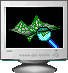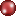"Excited polaron states in condensed media""Polarons & Applications"Publications on the subject

The mathematical object of investigation in LQS is the study on a class of multiparameter nonlinear boundary value problems for the system of integrodifferential equations modeling critical regimes in physical and biological proceses.
The objects of research are:
- The spectral problem for the system of integro-differential equations
 (A)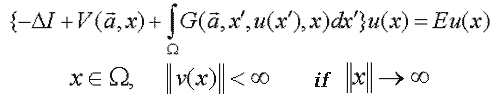- The joint systems for the nonlinear boundary value problem (B) and the spectral problem (C)
 (B)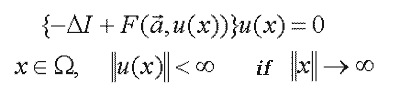(C)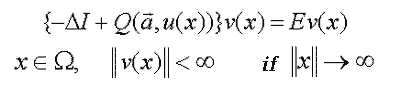where :
W - is a domain of х with indefinite boundary;
a - is the vector of the model's physical parameters;
E - is the spectral parameter (E=E(a));
I - is the unit operator.

The above mentioned problems represent a new class of mathematical objects with some common characteristics such as non-linearity, singularity, multiparametricity on external physical parameters, multidimensionality of configuration space and nonuniquehess of solutions. On the whole, the enumerated problems can be characterized as a class of nonlinear problems, wich describes the evolution of complicated systems with bifurcations and critical regimes.
Some examples of (A), (B) and (C) are represented in this section.

1. Equation f3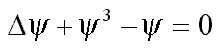1.1. Spherically-symmetric solutions in R3 ( )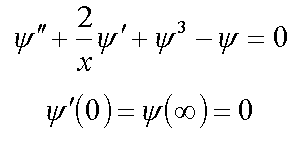1.2. Spherically-symmetric solutions in R2 ( )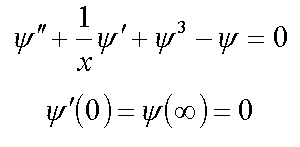1.3. Spherically-unsymmetric solutions ( )

2. Strong-coupling polaron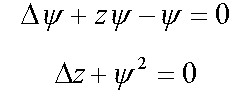2.1. Spherically-symmetric solutions in R3 ( , )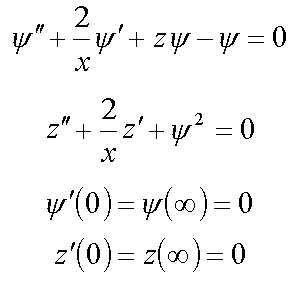2.2. . Spherically-unsymmetric solutions ( , )

3. Equation for bound condenson ( )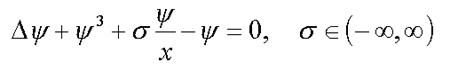3.1. Spherically-symmetric solutions in R3 ( )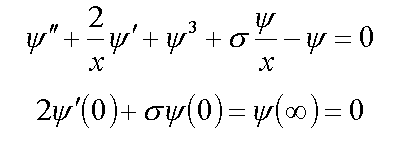3.2. Spherically-unsymmetric solutions

4. Equation for F-center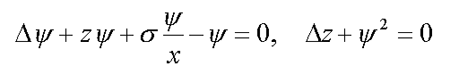4.1. Spherically-symmetric solutions in R3 ( , )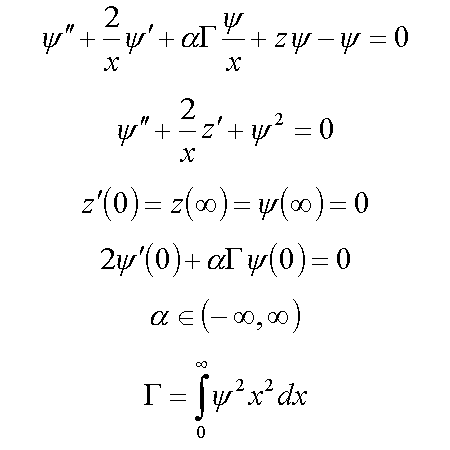4.2. Spherically-unsymmetric solutions ( , )

5. Polaronic condenson ( )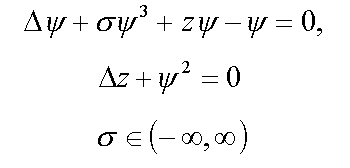6. Bound polaronic condenson ( )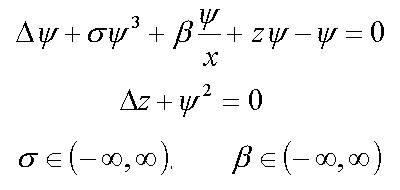7. Electron in disordered medium with ( )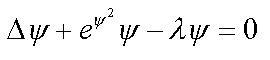7.1. Spherically-symmetric solutions in R3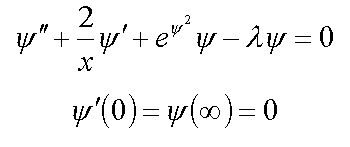8. Electron in disordered medium with repulsoin potential ( )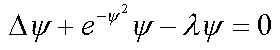1. Spherically-symmetric solutions in R3 ( )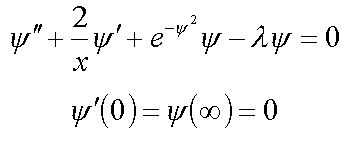9. Continuum exciton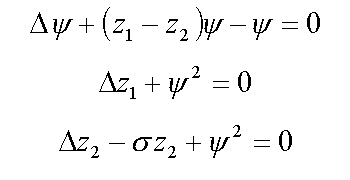9.1. Spherically-symmetric solutions in R3 ( , )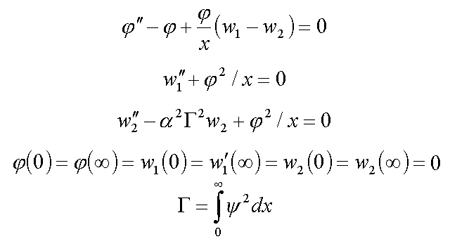9.2. Spherically-unsymmetric solutions in R3 ( )

10. Continuum biexciton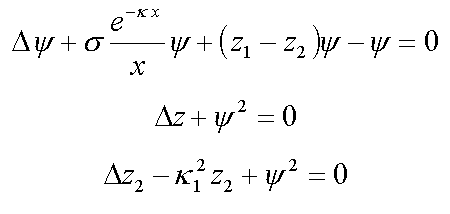10.1. Spherically-symmetric solutions ( , )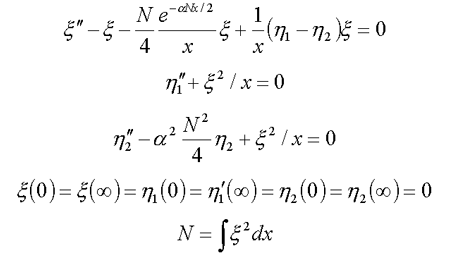10.2. Spherically-unsymmetric solutions

11. Solvated electron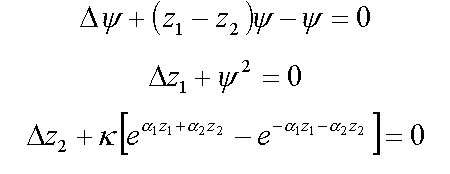11.1. Spherically-symmetric case ( )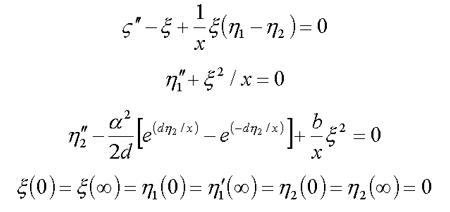12. Nucleon in scalar meson field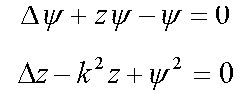12.1. Spherically-symmetric solutions in R3 ( , )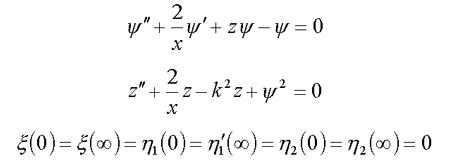12.2. Spherically-unsymmetric solutions in R2 ( )
12.3. Spherically-symmetric solutions for nucleon in the nucleus ( )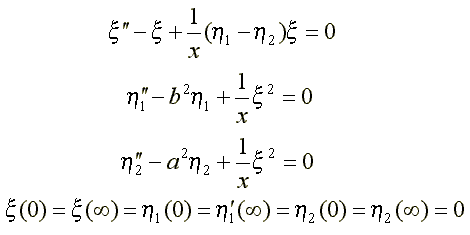13. Bipolaronic exciton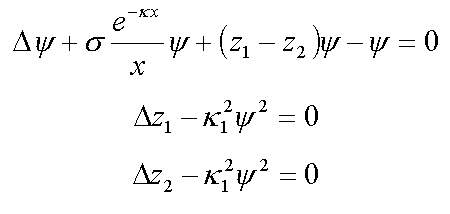14. Nonlinear equations of electron extended ststes in proteins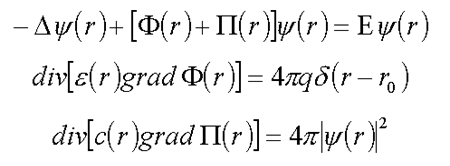Functions e(r), c(r) are predetermined ( ).

15. Equationa for particles in a quantum field at arbitrary strength of coupling ( )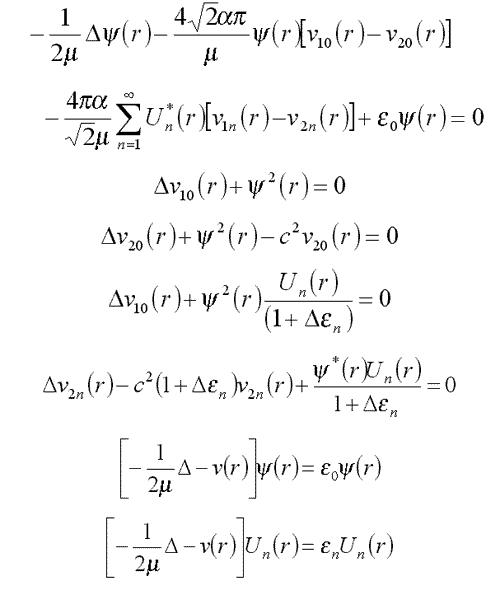16. Equations for fluctuons
16.1. General case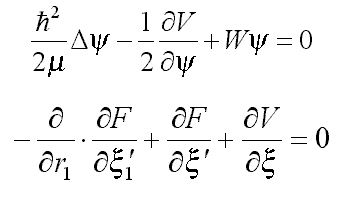16.2. In the context applicability of Landau-Ginzburg-Devonshire (LGD) thermodynamic expansion (9.1) in the spherically-symmetric case (16.1) has the form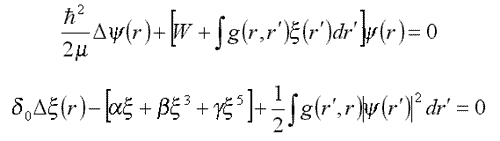where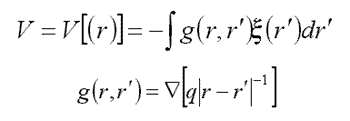Fluctuon for small homophase fluctuations: g(r,r')=g0(r-r').
F[x]=ax2
leads to the equation for deuteron (12.1).
16.3. Krivoglaz fluctuon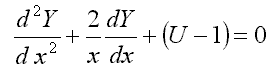where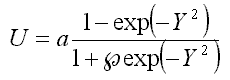.
17. Discrete models
17.1. Electron in DNA ( , )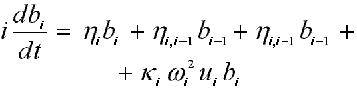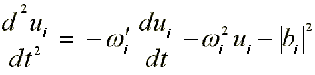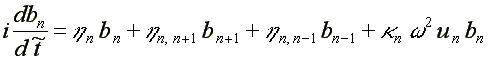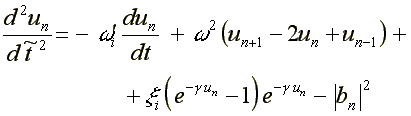where: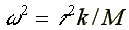17.2. Electron in the photoreaction center of the photosyntesis ( , )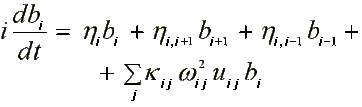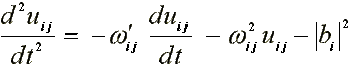--------------------------------------
1* - R.Friedberg, J.M.Luttinger. Density of electornic levels in disordered systems //Phys. Rev. B., 1975, v.12, №10, p.4460-4474
2* - J.Wisem. Nonlinear waves. - Moscow : Mir, 1977 ( in Russian )
3* - G.L.Alfimov, V.M.Eleonsky, N.E.Kulagin, L.M.Lerman, V.P.Silin. Two-dimensional self-localized solutions of the equation Du-u+u3=0 : Preprint №238. - Moscow : Physical Institute of the USSR Academy of Sciences, 1988, p.27 ( in Russian )
4* - A.S.Davydov. Solid state theory. - Moscow : Nauka, 1976 ( in Russian )
5* - K.A.Gorshkov, V.A.Mironov, A.M.Sergeev. Nonlinear waves, self-organization. - Moscow : Nauka, 1983, с.112-128 ( in Russian )
6* - I.M.Livshits, S.A.Gredeskul, L.A.Pastur. Introduction into the theory of disordered systems. - Moscow : Nauka, 1982 ( in Russian )
7* - I.V.Amirkhanov, I.V.Puzynin, T.P.Puzynina, E.V.Zemljanaja. Iteration Method for Solving the Spherical Non-symmetrical Polaron Equation (The Luttinger LU Model) // / Ed. V.D.Lakhno. - Leeds: J.Wiley & Sons Ltd, 1994, p.445-453"Excited polaron states in condensed media""Polarons & Applications"Publications on the subject